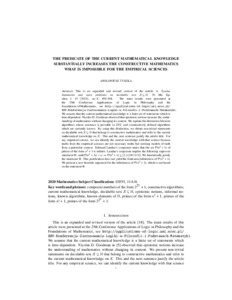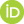# The predicate of the current mathematical knowledge substantially increases the constructive mathematics what is impossible for the empirical sciences

Tyszka, Apoloniusz (2023) The predicate of the current mathematical knowledge substantially increases the constructive mathematics what is impossible for the empirical sciences. [Preprint]There is a more recent version of this item available.Preview
Text
a_tyszka_july_27.pdf

## Abstract

This is an expanded and revised version of the article: A. Tyszka, Statements and open problems on decidable sets X⊆N, Pi Mu Epsilon J. 15 (2023), no. 8, 493-504. The main results were presented at the 25th Conference Applications of Logic in Philosophy and the Foundations of Mathematics, see http://applications-of-logic.uni.wroc.pl/XXV-Konferencja-Zastosowania-Logiki-w-Filozofii-i-Podstawach-Matematyki. We assume that the current mathematical knowledge is a finite set of statements which is time-dependent. Nicolas D. Goodman observed that epistemic notions increase the understanding of mathematics without changing its content. We explain the distinction between algorithms whose existence is provable in ZFC and constructively defined algorithms which are currently known. By using this distinction, we obtain non-trivial statements on decidable sets X⊆N that belong to constructive mathematics and refer to the current mathematical knowledge on X. This and the next sentence justify the article title. For any empirical science, we can identify the current knowledge with that science because truths from the empirical sciences are not necessary truths but working models of truth from a particular context. Edmund Landau's conjecture states that the set P(n^2+1) of primes of the form n^2+1 is infinite. Landau's conjecture implies the following unproven statement Φ: card(P(n^2+1))<ω ⇒ P(n^2+1)⊆[2,(((24!)!)!)!]. We heuristically justify the statement Φ. This justification does not yield the finiteness/infiniteness of P(n^2+1). We present a new heuristic argument for the infiniteness of P(n^2+1), which is not based on the statement Φ.

 Export/Citation: EndNote | BibTeX | Dublin Core | ASCII/Text Citation (Chicago) | HTML Citation | OpenURL
 Social Networking:

Item Type: Preprint
Creators:
CreatorsEmailORCIDTyszka, Apoloniuszrttyszka@cyf-kr.edu.pl0000-0002-2770-5495
Keywords: composite numbers of the form 2^{2^n}+1, constructive algorithms, current mathematical knowledge, decidable sets X⊆N, epistemic notions, informal notions, known algorithms, known elements of N, primes of the form n^2+1, primes of the form n!+1, primes of the form 2^{2^n}+1
Subjects: Specific Sciences > Mathematics > Epistemology
Specific Sciences > Mathematics
Depositing User: Apoloniusz Tyszka
Date Deposited: 28 Jul 2023 17:55
Item ID: 22337
Official URL: http://applications-of-logic.uni.wroc.pl/XXV-Konfe...
Subjects: Specific Sciences > Mathematics > Epistemology
Specific Sciences > Mathematics
Date: 27 July 2023
URI: http://philsci-archive.pitt.edu/id/eprint/22337

### Monthly Views for the past 3 yearsView Item